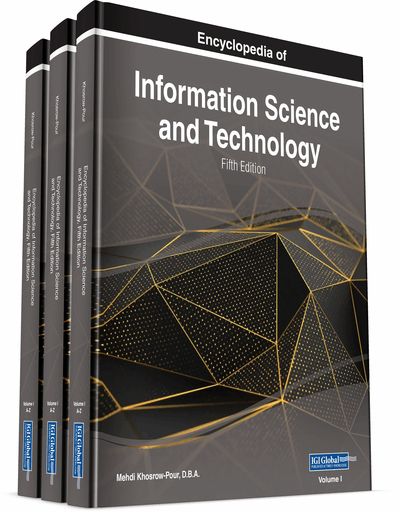# A General-Purpose Uniform Random Number Package

DOI: 10.4018/978-1-7998-3479-3.ch013
OnDemand:
(Individual Chapters)
Available
\$37.50
No Current Special Offers

## Abstract

As computer capacities and simulation technologies advance, simulation has become the method of choice for modeling and analysis. The fundamental advantage of simulation is that it can tolerate far less restrictive modeling assumptions, leading to an underlying model that is more reflective of reality and thus more valid, leading to better decisions. Simulation studies are typically preceded by transforming in a more or less complicated way of a sequence of numbers between 0 and 1 produced by a pseudorandom generator into an observation of the measure of interest. Random numbers are a fundamental resource in science and technology. A facility for generating sequences of pseudorandom numbers is a fundamental part of computer simulation systems. Furthermore, random number generators also play an important role in cryptography and in the blockchain ecosystem. All samples of the sequence are generated independently of each other, and the value of the next sample in the sequence cannot be predicted, regardless of how many samples have already been produced.
Chapter Preview
Top

## Background

There are several methods for generating the random numbers, of which the most popular are the congruential methods (mixed, multiplicative, and additive). These congruential methods are fast, convenient, and have good enough statistical properties when their parameters are chosen carefully. The (mixed) linear congruential generators (LCGs) are defined by

where m (the modulus) is a positive integer (usually a very large primary number), a (the multiplier) ∈{0,1,…,m–1} and c (the increment) is a nonnegative integer. This mathematical notation signifies that xi is the remainder ofdivided by m.. Hence, xi∈{0,1,…,m–1}. Thus, random variable ui is a uniform 0, 1 variable. Note that

## Key Terms in this Chapter

Blockchain: A blockchain is a growing list of digital records of transactions, called blocks, which are linked together using cryptograph. Each block contains a cryptographic hash of the previous block, a timestamp and transaction data.

Jump Ahead (Back) v Steps: A utility to advance (go back) v steps in the random number stream without going through the stream sequentially.

Variance Reduction: A technique to reduce the variance of the estimate, hence, increase the precision of the estimate.

Deterministic Algorithm: An algorithm that given the same input always products the same outputs.

Deterministic Sequence: A sequence that the value of any future elements can be determined when the value of the current element is known

Period Length (of RNGs): The length of the random number sequence before it recycles itself.

Quantile Function (Inverse Distribution Function): The quantile function (given a probability p ) returns the value at or below which 100 p percent of the population lies.

Common Random Numbers: A variance reduction technique that uses the same random number streams to simulation different systems.

Random Number Generators (RNGs): Computational or physical devices designed to generate a sequence of numbers that appear to be random, as determined by statistical tests.

## Complete Chapter List

Search this Book:
Reset